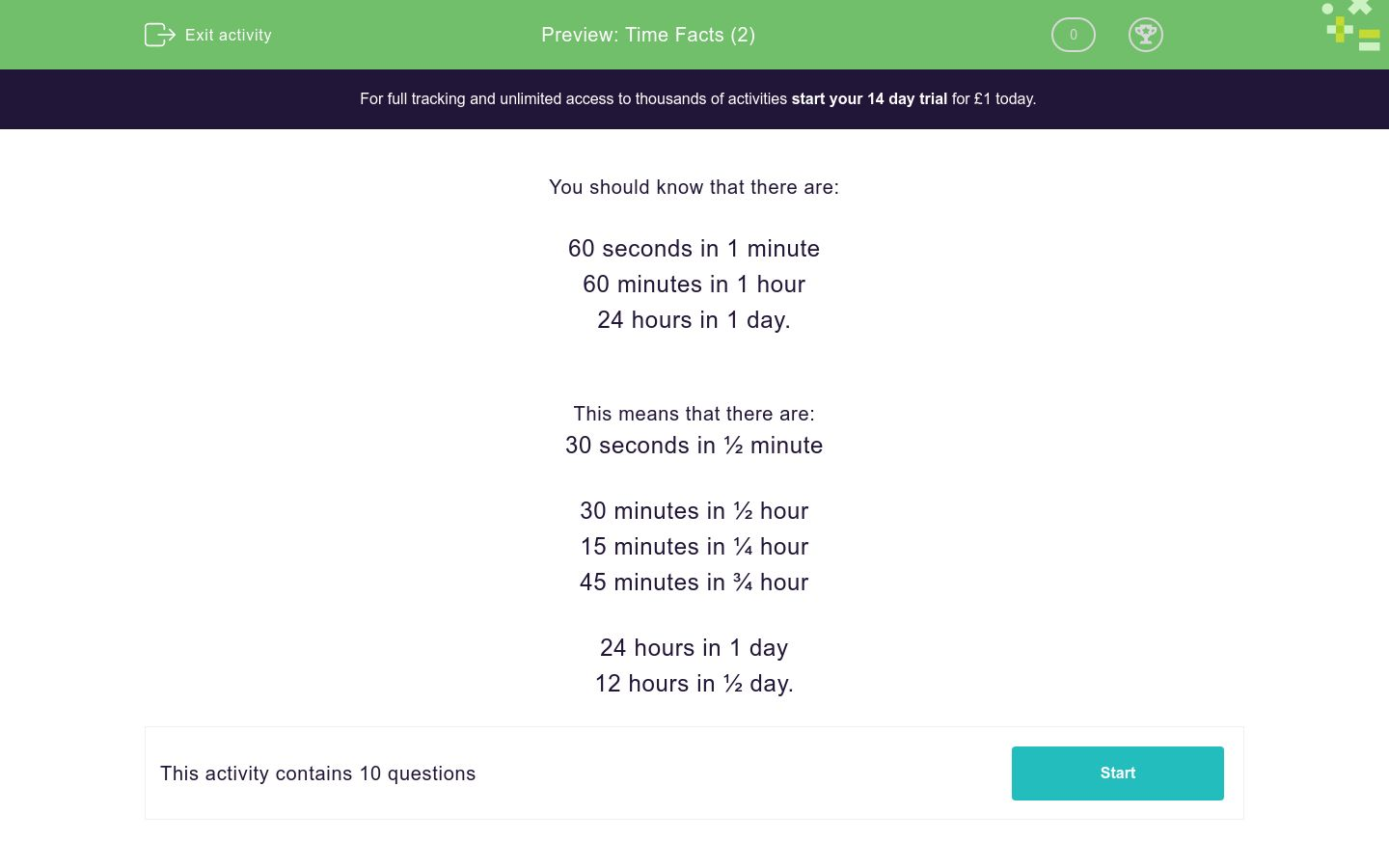# Time Facts (2)

In this worksheet, students must know how many seconds, minutes and hours there are in various time periods, including halves and quarters.Key stage:  KS 1

Curriculum topic:   Measurement

Curriculum subtopic:   Know Divisions of Time

Difficulty level:### QUESTION 1 of 10

You should know that there are:

60 seconds in 1 minute

60 minutes in 1 hour

24 hours in 1 day.

This means that there are:

30 seconds in ½ minute

30 minutes in ½ hour

15 minutes in ¼ hour

45 minutes in ¾ hour

24 hours in 1 day

12 hours in ½ day.

Write the missing number below:

There are ______ minutes in 1 hour.

Write the missing number below:

There are ______ seconds in 1 minute.

Write the missing number below:

There are ______ hours in half a day.

Write the missing number below:

There are ______ seconds in 2 minutes.

Write the missing number below:

There are ______ seconds in ½ minute.

Write the missing number below:

There are ______ minutes in ½ hour.

Complete:

There are 30 seconds in ______ minute.

a whole

half a

a quarter of a

Complete:

There are 15 minutes in ______ hour.

a whole

half an

a quarter of an

Write the missing number below:

There are ______ minutes in ¾ hour.

Complete:

There are 120 seconds in ______ minutes.

• Question 1

Write the missing number below:

There are ______ minutes in 1 hour.

60
EDDIE SAYS
60 minutes = 1 hour
• Question 2

Write the missing number below:

There are ______ seconds in 1 minute.

60
EDDIE SAYS
60 seconds = 1 minute
• Question 3

Write the missing number below:

There are ______ hours in half a day.

12
EDDIE SAYS
24 hours = 1 day
• Question 4

Write the missing number below:

There are ______ seconds in 2 minutes.

120
EDDIE SAYS
60 seconds = 1 minute
• Question 5

Write the missing number below:

There are ______ seconds in ½ minute.

30
EDDIE SAYS
60 seconds = 1 minute
• Question 6

Write the missing number below:

There are ______ minutes in ½ hour.

30
EDDIE SAYS
60 minutes = 1 hour
• Question 7

Complete:

There are 30 seconds in ______ minute.

half a
EDDIE SAYS
60 seconds = 1 minute
• Question 8

Complete:

There are 15 minutes in ______ hour.

a quarter of an
EDDIE SAYS
60 minutes = 1 hour
• Question 9

Write the missing number below:

There are ______ minutes in ¾ hour.

45
EDDIE SAYS
¼ hour = 15 mins so ¾ hour = 45 mins
• Question 10

Complete:

There are 120 seconds in ______ minutes.

2
EDDIE SAYS
60 seconds = 1 minute
---- OR ----

Sign up for a £1 trial so you can track and measure your child's progress on this activity.

### What is EdPlace?

We're your National Curriculum aligned online education content provider helping each child succeed in English, maths and science from year 1 to GCSE. With an EdPlace account you’ll be able to track and measure progress, helping each child achieve their best. We build confidence and attainment by personalising each child’s learning at a level that suits them.

Get started